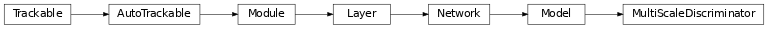MultiScaleDiscriminator¶

Inheritance Diagramclass ashpy.models.convolutional.discriminators.MultiScaleDiscriminator(input_res, min_res, kernel_size, initial_filters, filters_cap, use_dropout=True, dropout_prob=0.3, non_linearity=<class 'tensorflow.python.keras.layers.advanced_activations.LeakyReLU'>, normalization_layer=<class 'ashpy.layers.instance_normalization.InstanceNormalization'>, use_attention=False, n_discriminators=1)[source]

Bases: tensorflow.python.keras.engine.training.Model

Multi-Scale discriminator.

This discriminator architecture is composed by multiple discriminators working at different scales. Each discriminator is a ashpy.models.convolutional.discriminators.PatchDiscriminator.

Examples

x = tf.ones((1, 256, 256, 3))

# instantiate the PathDiscriminator
multiScaleDiscriminator = MultiScaleDiscriminator(input_res=256,
min_res=16,
kernel_size=5,
initial_filters=64,
filters_cap=512,
n_discriminators=3
)

# evaluate passing x
outputs = multiScaleDiscriminator(x)

# the output shape is
# the same as the input shape
print(len(outputs))
for output in outputs:
print(output.shape)
3
(1, 12, 12, 1)
(1, 12, 12, 1)
(1, 12, 12, 1)

Methods

 __init__(input_res, min_res, kernel_size, …) Multi Scale Discriminator. build_discriminator(input_res) Build a single discriminator using parameters defined in this object. call(inputs[, training, return_features]) Forward pass of the Multi Scale Discriminator.

Attributes

 activity_regularizer Optional regularizer function for the output of this layer. dtype dynamic inbound_nodes Deprecated, do NOT use! Only for compatibility with external Keras. input Retrieves the input tensor(s) of a layer. input_mask Retrieves the input mask tensor(s) of a layer. input_shape Retrieves the input shape(s) of a layer. input_spec Gets the network’s input specs. layers losses Losses which are associated with this Layer. metrics Returns the model’s metrics added using compile, add_metric APIs. metrics_names Returns the model’s display labels for all outputs. name Returns the name of this module as passed or determined in the ctor. name_scope Returns a tf.name_scope instance for this class. non_trainable_variables non_trainable_weights outbound_nodes Deprecated, do NOT use! Only for compatibility with external Keras. output Retrieves the output tensor(s) of a layer. output_mask Retrieves the output mask tensor(s) of a layer. output_shape Retrieves the output shape(s) of a layer. run_eagerly Settable attribute indicating whether the model should run eagerly. sample_weights state_updates Returns the updates from all layers that are stateful. stateful submodules Sequence of all sub-modules. trainable trainable_variables Sequence of variables owned by this module and it’s submodules. trainable_weights updates variables Returns the list of all layer variables/weights. weights Returns the list of all layer variables/weights.
__init__(input_res, min_res, kernel_size, initial_filters, filters_cap, use_dropout=True, dropout_prob=0.3, non_linearity=<class 'tensorflow.python.keras.layers.advanced_activations.LeakyReLU'>, normalization_layer=<class 'ashpy.layers.instance_normalization.InstanceNormalization'>, use_attention=False, n_discriminators=1)[source]

Multi Scale Discriminator.

Different generator for different scales of the input image.

Used by Pix2PixHD  .

Parameters: input_res (int) – input resolution min_res (int) – minimum resolution reached by the discriminators kernel_size (int) – kernel size of discriminators initial_filters (int) – number of initial filters in the first layer of the discriminators filters_cap (int) – maximum number of filters in the discriminators use_dropout (bool) – whether to use dropout dropout_prob (float) – probability of dropout non_linearity (tf.keras.layers.Layer) – non linearity used in discriminators normalization_layer (tf.keras.layers.Layer) – normalization used by the discriminators use_attention (bool) – whether to use attention n_discriminators (int) – Number of discriminators
  High-Resolution Image Synthesis and Semantic Manipulation with Conditional GANs https://arxiv.org/abs/1711.11585
build_discriminator(input_res)[source]

Build a single discriminator using parameters defined in this object.

Parameters: input_res – input resolution of the discriminator. Encoder A Discriminator (PatchDiscriminator).
call(inputs, training=True, return_features=False)[source]

Forward pass of the Multi Scale Discriminator.

Parameters: inputs (tf.Tensor) – input tensor. training (bool) – whether is training or not. return_features (bool) – whether to return features or not. Union[List[Tensor], Tuple[List[Tensor], List[Tensor]]] ([tf.Tensor]) – A List of Tensors containing the value of D_i for each input. ([tf.Tensor]): A List of features for each discriminator if return_features.